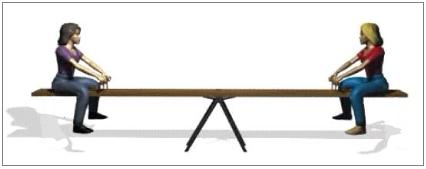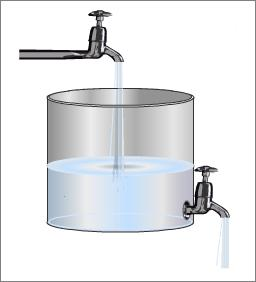Reversible and irreversible reactions

It is a common observation that most of the reactions when carried out in closed vessels do not go to completion, under a given set of conditions of temperature and pressure. In fact in all such cases, in the initial state, only the reactants are present but as the reaction proceeds, the concentration of reactants decreases and that of products increases. Finally a stage is reached when no further change in concentration of the reactants and products is observed.

Example 1

If a mixture of gaseous hydrogen and iodine vapors is made to react at 717 k in a closed vessel for about 2 - 3 hours, gaseous hydrogen iodide is produced according to the following equation:

$H_{2\; (g)} + I_{2\; (g)} \rightarrow 2HI_{(g)}$

But along with gaseous hydrogen iodide, there will be some amount of unreacted gaseous hydrogen and gaseous iodine left. On the other hand if gaseous hydrogen iodide is kept at 717K in a closed vessel for about 2 - 3 hours it decomposes to give gaseous hydrogen and gaseous iodine.

$2HI_{(g)} \rightarrow H_{2\; (g)} + I_{2\; (g)}$

In this case also some amount of gaseous hydrogen iodide will be left unreacted. This means that the products of certain reactions can be converted back to the reactants. These types of reactions are called reversible reactions.

Thus, in reversible reactions the products can react with one another under suitable conditions to give back the reactants. In other words, in reversible reactions the reaction takes place in both the forward and backward directions. The reversible reaction may be expressed as:

$H_{2\; (g)} + I_{2\; (g)} \rightleftharpoons 2HI_{(g)}$

These reversible reactions never go to completion if performed in a closed container. For a reversible chemical reaction, an equilibrium state is attained when the rate at which a chemical reaction is proceeding in forward direction equals the rate at which the reverse reaction is proceeding.

Equilibrium

This state at which the concentration of reactants and products do not change with time is called a state of chemical equilibrium. The amount of reactants unused depend on the experimental conditions such as concentration, temperature, pressure and the nature of the reaction. At equilibrium, the rate of forward reaction is equal to the rate of reverse reaction

Consider the reversible reaction

$N_{2\; (g)} + 3H_{2\; (g)} \rightleftharpoons 2NH_{3\; (g)}$

When this reaction is performed at high pressure and temperature in a close container, at equilibrium,

Rate of formation of ammonia = Rate of decomposition of ammonia

Now, the question arises whether all the ammonia molecules are remaining intact and not decomposing? Are all the molecules of nitrogen and hydrogen becoming inactive and not combining? If were is the case, we would say a static equilibrium is attained.Figure 1: Static Equilibrium with no motion (no dynamics) occurring

To understand the concept of static equilibrium, let us consider two children sitting on a see-saw. At balance point (i.e., the equilibrium position) no movement of children on the see-saw occurs.

In the case of reversible reactions, however a static equilibrium is not being established.

In the case of ammonia, using deuterium, $$D$$ (an isotope of hydrogen) it has been proved that even at equilibrium, decomposition of ammonia into hydrogen and nitrogen and combination of hydrogen and nitrogen into ammonia continues. This equilibrium is dynamic in nature and is therefore called dynamic equilibrium.Figure 2: Dynamic Equilibrium with motion (dynamics) occurring via flow in and flow out

A dynamic steady state can be compared with the equilibrium of water in a reservoir, which is being simultaneously filled and discharged. If the rate of water flowing in is equal to the rate of water flowing out, the quantity of water in the reservoir will remain unchanged like the quantities of substances in a state of chemical equilibrium. Since the rate of water entering is equal to the rate of water leaving, the level of water is constant

Similarly, some other examples of reversible reactions are:

$N_{2\; (g)} + O_{2\; (g)} \rightleftharpoons 2NO_{(g)}$

$2SO_{2\; (g)} + O_{2\; (g)} \rightleftharpoons 2SO_{3\;(g)}$

On the other hand, the chemical reaction in which the products formed do not combine to give the reactants are known as irreversible reactions.

For e.g., potassium chlorate decomposes on heating to form potassium chloride and oxygen.

$2KClO_{3\;(s)} \overset{\Delta}{\rightarrow} 2KCl_{(s)} + 3O_{2\;(g)}$

However the products cannot combine to form potassium chlorate. In case of irreversible physical and chemical processes, the change occurs only in one direction and the processes go to completion. However, the reversible processes do not go to completion and appear to stop (attain state of dynamic equilibrium) with some starting materials are remaining (although constantly being consumed and produced).

Some examples of irreversible reactions include the precipitation of silver chloride from solution:

$AgNO_{3\;(aq)} + NaCl_{(aq)} \rightarrow AgCl_{(s)}+ NaNO_{3\; (s)}$

and the combustion of magnesium

$2Mg_{(s)} + O_{2\; (g)} \rightarrow 2MgO_{(s)}$

It may be noted that for reversible reactions the symbol $$\rightleftharpoons$$ is used between the reactants and products. For irreversible reactions, single headed arrow $$\rightarrow$$ is used.

Contributors

Binod Shrestha (University of Lorraine)